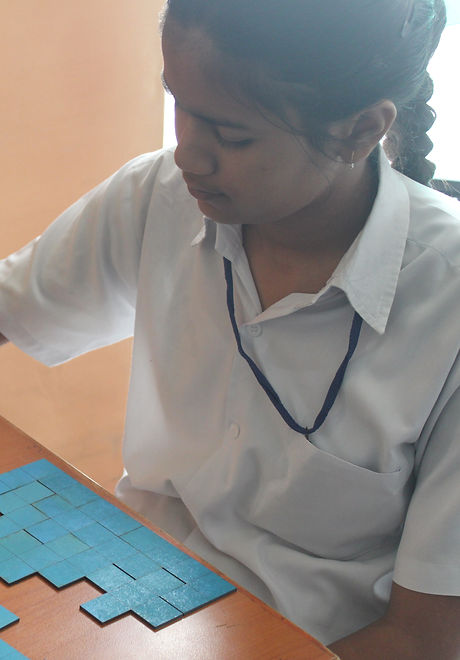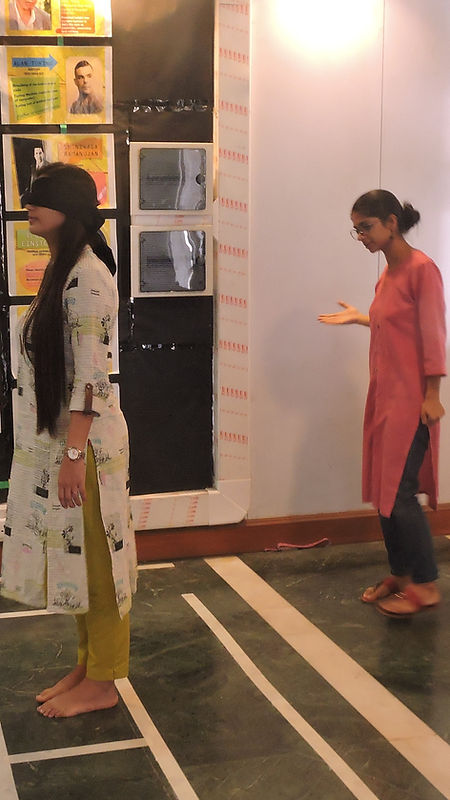# Learning Resources

Find various resources for Mathematics Education here.

## GeoGebra Applets Library

GeoGebra is an interactive geometry, algebra, statistics and calculus application, intended for learning and teaching mathematics and science from primary school to university level.

This is the library of several GeoGebra Applets created by the students for different math concepts.## Pentominoes Puzzle

A pentomino is a polygon in the plane made of 5 equal-sized squares connected edge-to-edge.  Pentomino puzzles require the learner to assemble the set into a larger given shape without overlaps.  Some examples are arranging the set of pentominoes to form rectangles of dimensions 12 by 5, 10 by 6, 15 by 4, 20 by 6.

Creators: Robin Sharma and Sourabh Garg## Dimension Destination (physical game)

This game is played by a team of 2 players at a time. One player is blind-folded and has to find their way through a maze. The other player communicates the path to them but can only use mathematical terminology and vocabulary. The team which takes the minimum amount of time wins. The objective of the game is to promote mathematical communication.

Creators: Anjali Thakran, Ishmannan Kaur, and Shilpa Goel

-## Exponent Tool

This tool deals with the concept of exponents. It helps in visualizing the concept using blocks that can be easily manipulated. For example, to visualize 3^1, one can show 3 blocks at a time and for 3^2, one will take 3 blocks three times which will be 9 blocks together. This way one can go on up to certain numbers and using this a child can visualize how repeated multiplication works.

Creators: Anjali Thakran, Ishmannan Kaur, and Shilpa Goel

-This tool enables the learner to verify the angle sum property of cyclic quadrilaterals for an infinite number of quadrilaterals formed by taking vertices on a fixed circle. The learner can move the vertices of the quadrilateral along the circle and measure the angles formed at the vertices using the 360-degree protractors attached at the intersection of two sides. It can also be used to verify other properties related to cyclic quadrilaterals like the relationship between the exterior angles, the relationship between opposite angles, etc.

Creators: Adeeba Samreen, Syed Shahzar, and Uzma Masood

-## Circular Track

This tool enables the learner to verify the angle sum property of cyclic quadrilaterals for an infinite number of quadrilaterals formed by taking vertices on a fixed circle. The learner can move the vertices of the quadrilateral along the circle and measure the angles formed at the vertices using the 360-degree protractors attached at the intersection of two sides. It can also be used to verify other properties related to cyclic quadrilaterals like the relationship between the exterior angles, the relationship between opposite angles, etc.

Creators: Adeeba Samreen, Syed Shahzar, and Uzma Masood

-## Basic Proportionality Theorem Tool

This tool enables the learner to verify the Basic Proportionality Theorem (BPT) for some combinations of measurements of sides of a triangle. The learner can move the segment parallel to one side of the triangle to change its intersection points with the other two sides, measure the sides and verify if the BPT holds true or not.

-